Next: Laplace's Equation in Spherical Up: Potential Theory Previous: Associated Legendre Functions

Spherical Harmonics

The spherical harmonics,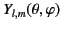, are the angular portions of the global solutions to Laplace's equation in standard spherical coordinates,,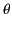,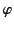. Here,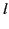is a non-negative integer (known as the degree), andis an integer (known as the order) lying in the range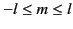. The spherical harmonics are well behaved and single valued functions that satisfy the differential equation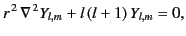(307)

and take the form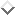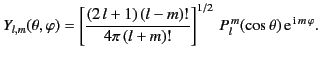(308)

It follows from Equation (295) that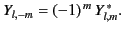(309)

Thesatisfy the orthonormality constraint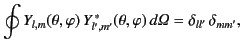(310)

where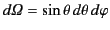is a an element of solid angle, and the integral is taken over all solid angle. Note that the spherical harmonics form a complete set of angular functions.

All of the spherical harmonics of degree less than 3 are listed below: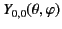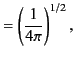(311)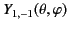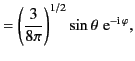(312)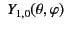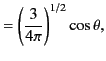(313)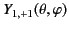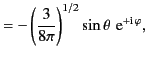(314)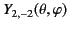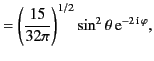(315)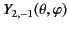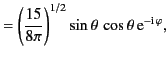(316)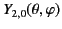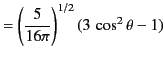(317)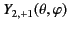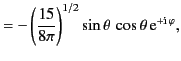(318)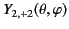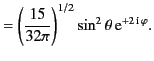(319)

Consider two spherical coordinate systems,,,and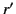,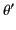,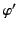, whose origins coincide, but whose polar axes subtend an angle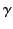with respect to one another. It follows that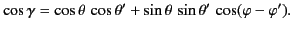(320)

Moreover, the so-called addition theorem for spherical harmonics states that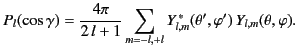(321)Next: Laplace's Equation in Spherical Up: Potential Theory Previous: Associated Legendre Functions
Richard Fitzpatrick 2014-06-27